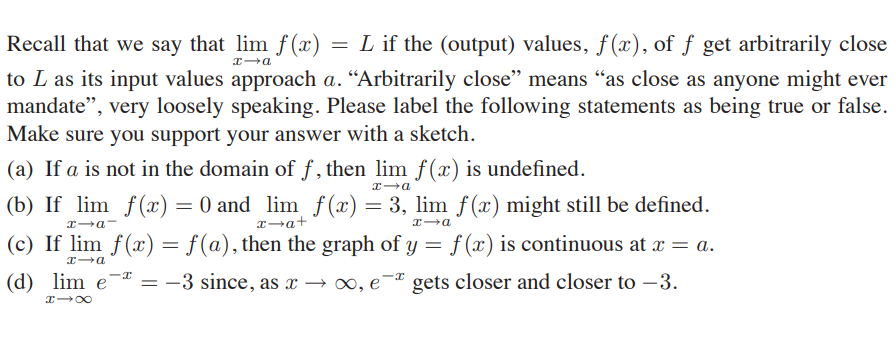# Recall that we say that lim f (x) = L if the (output) values, f(x), of ƒ get arbitrarily close to L as its input values approach a. “Arbitrarily close" means "as close as anyone might ever mandate", very loosely speaking. Please label the following statements as being true or false. Make sure you support your answer with a sketch. (a) If a is not in the domain of f , then lim f(x) is undefined. (b) If lim f() = 0 and lim f(x) = 3, lim f (x) might still be defined. x-a+ (c) If lim f(x) = f(a), then the graph of y = f (x) is continuous at x = a. %3D

Questionhelp_outlineImage TranscriptioncloseRecall that we say that lim f (x) = L if the (output) values, f(x), of ƒ get arbitrarily close to L as its input values approach a. “Arbitrarily close" means "as close as anyone might ever mandate", very loosely speaking. Please label the following statements as being true or false. Make sure you support your answer with a sketch. (a) If a is not in the domain of f , then lim f(x) is undefined. (b) If lim f() = 0 and lim f(x) = 3, lim f (x) might still be defined. x-a+ (c) If lim f(x) = f(a), then the graph of y = f (x) is continuous at x = a. %3D fullscreen

### Want to see this answer and more?

Experts are waiting 24/7 to provide step-by-step solutions in as fast as 30 minutes!*

*Response times may vary by subject and question complexity. Median response time is 34 minutes for paid subscribers and may be longer for promotional offers.
Tagged in
MathCalculus

### Limits